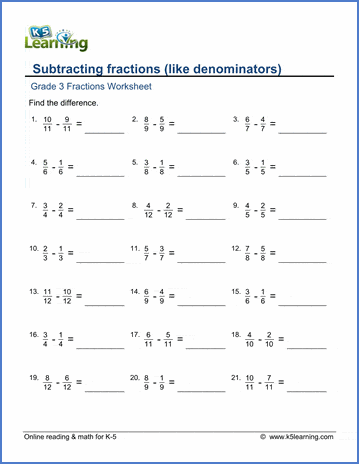# Subtracting fractions with like denominators

## Fractions worksheet: Subtracting proper fractions with like denominators

Below are six versions of our grade 3 math worksheet on subtracting fractions with the same denominators.  All answers are positive, proper fractions.  Denominators are between 2 and 12.  These worksheets are pdf files.## More fractions worksheets

Explore all of our fractions worksheets, from dividing shapes into "equal parts" to multiplying and dividing improper fractions and mixed numbers.

## What is K5?

K5 Learning offers reading and math worksheets, workbooks and an online reading and math program for kids in kindergarten to grade 5.  We help your children build good study habits and excel in school.